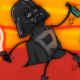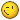# My take on a 3D stereoscopic shader

• NotWorthy
•Topic Author
• Offline
3 years 1 week ago #1 by NotWorthy
My take on a 3D stereoscopic shader was created by NotWorthy

Ok I'm gonna keep this short since for one thing I hear 3D is dying and secondly because I see someone else already mastered the topic here.
So I made this very simple shader that nicely generates a would-like 3D image from the depthmap at-hand - filling the blanks with a twean of sorts that isnt very noticable - the artifacts around objects who are at contrast with something with a far different depth are a little bit noticable, but when you feel the distance between characters, buildings, mountains in the far - you let those artifacts slide. It should work on any game with the depthmap available - setting aside the various ways depth maps are calculated amoungst games - it costs about 1.5fps on a 1920x1080 screen.
I feel ashamed sharing my convoluted code with you guys but I feel the urge to contribute:

Here is the contents of NotWorthy3d.fx (and don't forget to call it that)
```#include "ReShade.fxh"

uniform float NW3D_ForcedDepth <
ui_type = "drag";
ui_min = 10.0; ui_max = 1000.0;
ui_tooltip = "Force More Depth into the effect.";
> = 88.0;

uniform float NW3D_Nest <
ui_type = "drag";
ui_min = 0.05; ui_max = 7.5;
ui_tooltip = "Falloffs starting point.";
> = 0.265;

uniform float NW3D_something4 <
ui_label = "Tweak Focus 1*2";
ui_type = "drag";
ui_min = -8.0; ui_max = 8.0;
ui_tooltip = "Combine Focus Tweak.";
> = 1.0;

uniform float NW3D_something1 <
ui_label = "Tweak Focus 1";
ui_type = "drag";
ui_min = 0.5; ui_max = 2.5;
ui_tooltip = "Focus tweak 1.";
> = 2.25;

uniform float NW3D_something3 <
ui_label = "Tweak Focus 2";
ui_type = "drag";
ui_min = -1.0; ui_max = 2.0;
ui_tooltip = "Focus tweak 2.";
> = 1.068;

uniform bool Reverse <
ui_label = "Reverse";
ui_tooltip = "Preview sharpen layer and mask for adjustment. If you don't see red strokes, try changing Preprocessor Definitions in the Settings tab.";
ui_category = "Debug View";
> = true;

uniform bool Preview <
ui_label = "Red/Cyan Glasses";
ui_tooltip = "Preview sharpen layer and mask for adjustment. If you don't see red strokes, try changing Preprocessor Definitions in the Settings tab.";
ui_category = "Debug View";
> = false;

texture texColorBufferX : COLOR;
texture texDepthBufferX : DEPTH;
sampler texColorBuffer { Texture = texColorBufferX;};
sampler BG { Texture = texColorBufferX;};
sampler texDepthBuffer { Texture = texDepthBufferX;};

float GetDD(float2 coords) {	return  tex2D(texDepthBuffer,coords.xy).x; }
float GetD(float2 coords) { return ((GetDD(coords))-0.45)*(sqrt(GetDD(coords))) + ((GetDD(coords))+0.45)*((3.0)-sqrt(3.0))  ; }

float sqr(float n){return n*n;}

float DD10(float Deepth, float Ratio) { return ((Deepth)-0.45)*sqrt(Deepth) + ((Deepth)+0.45)*(Ratio-sqrt(Deepth))  ; }
void PS_3D_A(float Nest, float InputRatio, float4 vpos : SV_Position, float2 Pos : TEXCOORD, out float4 Res : SV_Target0)
{
float Deepth = (GetD(Pos.xy));
float Deep = DD10(Deepth, InputRatio);
float Dept = 1.0-sqrt(abs(Deepth+NW3D_something1*NW3D_something4))*((Deepth)/abs(Deepth-0.0));
Deep = Deep*NW3D_something3*NW3D_something4+sqr(max(0.0,-Deep))*(1.0-Nest) + (Deep*0.75+sqrt(max(0.0,-Dept)))*Nest ;
Deep = Deep-46.0/0.4;
float row =((sign(frac((BUFFER_HEIGHT*Pos.y)/2.0)-0.5 )+1.0)/2.0);
float2 disp1 = float2(Pos.x+0.175*Deep*(Pos.x-0.1)-0.3*(max(-0.3,-Deep)+0.7)*Deep*(Pos.x-0.1)  ,Pos.y)*(1.0-Nest)+ float2(Pos.x+0.17*Dept*Deep*(Pos.x-0.1)-(0.325)*(max(-0.3,-Deep)+0.825)*Deep*(Pos.x-0.01)  ,Pos.y)*Nest;
float2 disp2 = float2(Pos.x+0.175*Deep*(Pos.x-0.9)-0.3*(max(-0.3,-Deep)+0.7)*Deep*(Pos.x-0.9)  ,Pos.y)*(1.0-Nest)+ float2(Pos.x+0.17*Dept*Deep*(Pos.x-0.9)-(0.325)*(max(-0.3,-Deep)+0.825)*Deep*(Pos.x-0.9)  ,Pos.y)*Nest;
float Reps1;
float Reps2;
Reps1=0.0;
Reps2=0.0;

[unroll]
while( DD10(GetD(disp1+(Pos-disp1)*float2(0.9-Reps1/16.0*0.9,0.0) ),InputRatio)*(1.0-Nest)+ DD10(GetD(disp1+(Pos-disp1)*float2(0.9-Reps1/16.0*0.9*Dept,0.0) ),InputRatio)*Nest > (Deep+sqrt(max(0.0,0.051-Deep*-0.1))*0.5)/0.75*(1.0-Nest) + (Deep+sqrt(max(0.0,0.051-Deep*-0.1))*0.5)/0.75*Dept*(Nest) && Reps1<16.0 ) { Reps1+=1.0;}// disp1.x+=(Pos.x-disp1.x)/1.25; }
[unroll]
while( DD10(GetD(disp2+(Pos-disp2)*float2(0.9-Reps2/16.0*0.9,0.0) ),InputRatio)*(1.0-Nest)+ DD10(GetD(disp2+(Pos-disp2)*float2(0.9-Reps2/16.0*0.9*Dept,0.0) ),InputRatio)*Nest > (Deep+sqrt(max(0.0,0.051-Deep*-0.1))*0.5)/0.75*(1.0-Nest) + (Deep*+sqrt(max(0.0,0.051-Deep*-0.1))*0.5)/0.75*Dept*(Nest) && Reps2<16.0 ) { Reps2+=1.0; }//disp2.x+=(Pos.x-disp2.x)/1.25; }

float orig = (0.45-min(0.45,Pos.x))*1 + (max(0.55,Pos.x)-0.55)*1;
disp1.x = Pos.x*orig + (disp1.x+(Pos.x-disp1.x)*(float(0.6-Reps1*Dept/16.0*0.6)*Nest+float(0.6-Reps1*Dept/16.0*0.6)*(1.0-Nest) ) )*(1.0-orig);
disp2.x = Pos.x*orig + (disp2.x+(Pos.x-disp2.x)*(float(0.6-Reps2*Dept/16.0*0.6)*Nest+float(0.6-Reps2*Dept/16.0*0.6)*(1.0-Nest) ) )*(1.0-orig);
float4 redcyan1 = float4(1.0,1.0,1.0,1.0);
float4 redcyan2 = float4(1.0,1.0,1.0,1.0);
if(Reverse)row=1.0-row;
if(Preview)
{
redcyan1 = float4(1.0,0.0,0.0,1.0);
redcyan2 = float4(0.0,1.0,1.0,1.0);
Res =    tex2D(BG, disp1)*redcyan1+ tex2D(BG, disp2)*redcyan2;
}
Res =    (1.0-row) * tex2D(BG, disp1)*redcyan1
+(0.0+row) * tex2D(BG, disp2)*redcyan2;
}

void NW_3D(float4 vpos : SV_Position, float2 Pos : TEXCOORD, out float4 Res : SV_Target0)
{
PS_3D_A(NW3D_Nest,NW3D_ForcedDepth, vpos, Pos, Res);
}

float BoundIt(float2 OPos, float2 BPos1, float2 BPos2)
{
float BIx = OPos.x-BPos1.x - (max(BPos2.x,OPos.x)-BPos2.x);
BIx = ((BIx+0.5)/abs(BIx+0.5)+1.0)/2.0;
float BIy = OPos.y-BPos1.y - (max(BPos2.y,OPos.y)-BPos2.y);
BIy = ((BIy+0.5)/abs(BIy+0.5)+1.0)/2.0;
return min((-sign(sign( (OPos.x)-BPos2.x )+0.5)+1.0)/2.0, (-sign(sign( (OPos.y)-BPos2.y )+0.5)+1.0)/2.0)
*(min((-sign(sign( BPos1.x-OPos.x )+0.5)+1.0)/2.0, (-sign(sign( BPos1.y-OPos.y )+0.5)+1.0)/2.0));
}

technique NotWorthy3D   {  pass NW3D
```
The following user(s) said Thank You: WalterDasTrevas

• Sami 1999
•• Offline
2 years 11 months ago #2 by Sami 1999
Replied by Sami 1999 on topic My take on a 3D stereoscopic shader
Only 1.5 FPS hit? That's a lot lower than forcing Nvidia 3d vision on games. I'm gonna give it a try.

•Topic Author